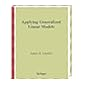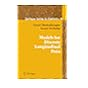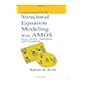Normal view

# Models for discrete longitudinal data / Geert Molenberghs, Geert Verbeke.

Material type:TextLanguage: English Publication details: c2006. Description: xxii, 683 p. : ill. 25 cmISBN: 0387251448 (hbk.); 9780387251448Subject(s): Multivariate analysis | Longitudinal methodDDC classification: 519.53 LOC classification: QA278 | .M65 2005Online resources: Publisher description | WorldCat details | Ebook Fulltext
Contents:
TOC Introduction -- Motivating studies -- Generalized linear models -- Linear mixed models for Gaussian longitudinal data -- Model families -- The strength of marginal models -- Likelihood-based marginal models -- Generalized estimating equations -- Pseudo-likelihood -- Fitting marginal models with SAS -- Conditional models -- Pseudo-likehood -- From subject-specific to random-effects models -- The generalized linear mixed model (GLMM) -- Fitting generalized linear mixed models with SAS -- Marginal versus random-effects models The analgesic trial -- Ordinal data -- The epilepsy data -- Non-linear models -- Pseudo-likelihood for a hierarchical model -- Random-effects models with serial correlation -- Non-Gaussian random effects -- Joint continuous and discrete responses -- High-dimensional joint models -- Missing data concepts -- Simple methods, direct likelihood, and WGEE -- Multiple imputation and the EM algorithm -- Selection models -- Pattern-mixture models -- Sensitivity analysis -- Incomplete data and SAS.
Summary: Summary: The linear mixed model is the main parametric tool for the analysis of continuous longitudinal data. This book shows how the different approaches can be implemented within the SAS software package.
Tags from this library: No tags from this library for this title.
Star ratingsAverage rating: 0.0 (0 votes)
Holdings
Item type Current library Collection Call number Copy number Status Date due Barcode Item holdsE-Book
E-book
Non-fiction 519.53 MOM 2006 (Browse shelf(Opens below)) Not for loanText
Reserve Section
Non-fiction 519.53 MOM 2006 (Browse shelf(Opens below)) C-1 Not For Loan 26628Text
Circulation Section
Non-fiction 519.53 MOM 2006 (Browse shelf(Opens below)) C-2 Available 26884Text
Circulation Section
Non-fiction 519.53 MOM 2006 (Browse shelf(Opens below)) C-3 Available 26885
Total holds: 0
##### Browsing EWU Library shelves, Shelving location: Circulation Section Close shelf browser (Hides shelf browser)519.53 LIA 1997 Applying generalized linear models / 519.53 LIA 1997 Applying generalized linear models / 519.53 MOM 2006 Models for discrete longitudinal data / 519.53 MOM 2006 Models for discrete longitudinal data / 519.53 THE 2013 Essentials of Monte Carlo simulation : 519.535 AGC 2013 Categorical data analysis / 519.535 BYS Structural equation modeling with AMOS :

Includes bibliographical references and index.

TOC Introduction --
Motivating studies --
Generalized linear models --
Linear mixed models for Gaussian longitudinal data --
Model families --
The strength of marginal models --
Likelihood-based marginal models --
Generalized estimating equations --
Pseudo-likelihood --
Fitting marginal models with SAS --
Conditional models --
Pseudo-likehood --
From subject-specific to random-effects models --
The generalized linear mixed model (GLMM) --
Fitting generalized linear mixed models with SAS --
Marginal versus random-effects models The analgesic trial --
Ordinal data --
The epilepsy data --
Non-linear models --
Pseudo-likelihood for a hierarchical model --
Random-effects models with serial correlation --
Non-Gaussian random effects --
Joint continuous and discrete responses --
High-dimensional joint models --
Missing data concepts --
Simple methods, direct likelihood, and WGEE --
Multiple imputation and the EM algorithm --
Selection models --
Pattern-mixture models --
Sensitivity analysis --
Incomplete data and SAS.

Summary:
The linear mixed model is the main parametric tool for the analysis of continuous longitudinal data. This book shows how the different approaches can be implemented within the SAS software package.

AS

Saifun Momota

There are no comments on this title.